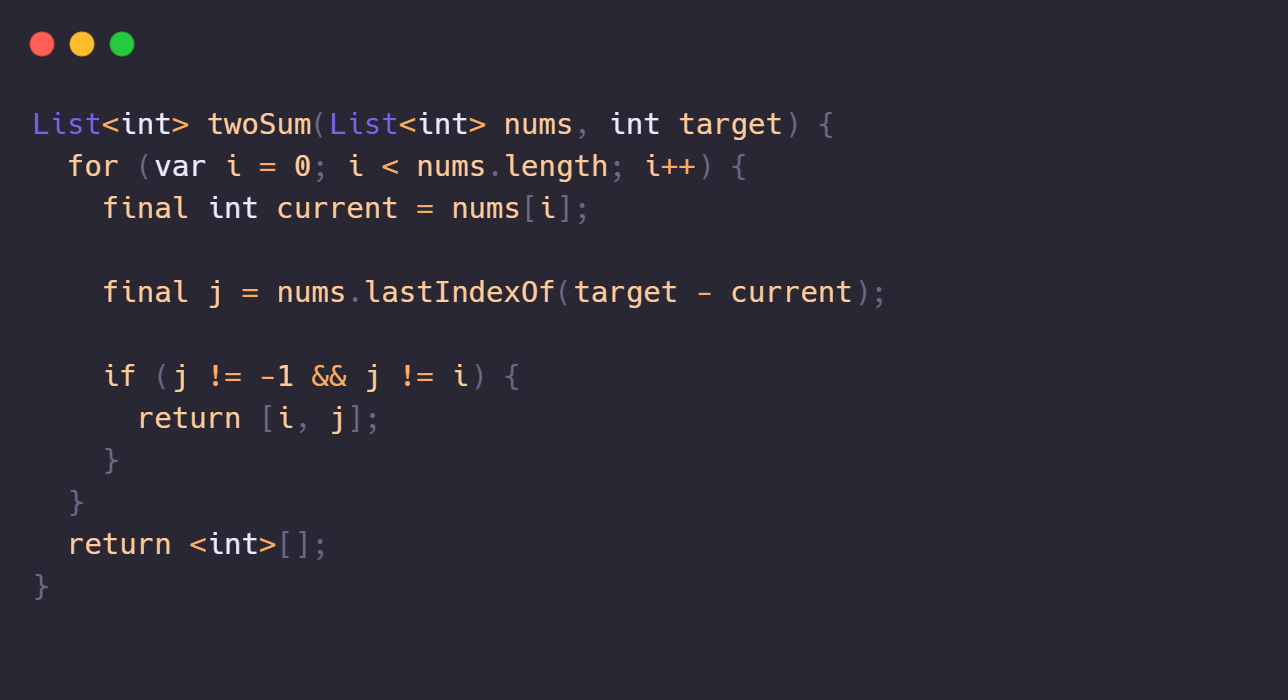By Alex Rintt (Last update November 30, 2022)

November 30, 2022Edit this file (Private, ask me for access if you wanna contribute)

# LeetCode, Two Sum problem solution using brute force in Dart

Two Sum LeetCode problem solution written in Dart in complexity O(N).Lets solve this problem from LeetCode: leetcode.com/problems/two-sum.

In the past I was addicted to algorithm exercises, in the old gold URI Online, what now is a preview of hell called Beecrowd, I need to write why I hate this website some time. But lets skip to what matters:

Given an array of N integers `nums<int>`, find the indices of two elements which the sum are equals to a given integer `target`.

Given an array of integers nums and an integer target, return indices of the two numbers such that they add up to target.

You may assume that each input would have exactly one solution, and you may not use the same element twice.

You can return the answer in any order.

Example 1:

Input: nums = [2,7,11,15], target = 9

Output: [0,1]

Explanation: Because nums + nums == 9, we return [0, 1].

Example 2:

Input: nums = [3,2,4], target = 6

Output: [1,2]

Example 3:

Input: nums = [3,3], target = 6

Output: [0,1]

The O(n²) is pretty simple: for each element of the array try to find a integer that summed is equals to the `target`.

``````List<int> twoSum(List<int> nums, int target) {
for (var i = 0; i < nums.length; i++) {
// Get the current iteration element.
final int current = nums[i];

final j = nums.lastIndexOf(target - current);

if (j != -1 && j != i) {
return [i, j];
}
}

// Never happens but due Dart null-safety you need to either throw an Exception or return the List<int>.
return <int>[];
}``````

The catch part is:

``nums.lastIndexOf(target - current);``

This can be described as a simple find x statement where x is the second sum element:

e = current element; x = second sum element; target = the total sum we are searching for.

x = target - e

Since we are done calculating the "what element should I search for as second element?" now we need to add a if statement to skip when we do not find a second element and when the second element is the first element itself ("and you may not use the same element twice."):

``````// If j == -1 then we didn't find a corresponding element.
// If j == i then the second element is the first element itself.
if (j != -1 && j != i) {
return [i, j];
}``````

Done, now we just return the `i` and `j` indices and submit our answer.

Challenge I wanna try next few days: find the optimized solution of this exercise; I'm not the dynamic programming guy yet, so I wanna Google and try to learn and understand, cheers me luck.

Files in the same folder:

• Donation - I accept donations from several platforms, this post serve as permalink to my documentation/credit pages for each project. These links allow supporters to easily find and donate to the projects they are interested in, while ensuring that all donations are properly credited and acknowledged.
• Creating a simple stargazing effect with p5.js - From a set of points, generate a stargazing effect.
• Acho que esse é um dos maiores crimes que já cometi - Criei um blog estático em NodeJS com puro HTML e CSS misturado com React e JavaScript + Babel, API, database, regra de negócio tudo em 1 único arquivo com 700 linhas.
• Qual a maneira mais simples de evoluir a si mesmo? - Se você já se perguntou, 'Como posso ser melhor?' em qualquer aspecto. Essa dica é definitivamente para você!
• Mr. Fear - Medo de aprender, medo de perguntar, medo de errar, medo de falar em público, medo de altura, medo de cruzar aquele beco num sábado a noite no centro da zona leste. Essas 4 letras não tem espaço suficiente pra agrupar essa complexa quantidade de sentimentos.
• Listar os últimos usuários que deram star em um repositório do GitHub - Com a API rest é impossível listar em order decrescente, portanto utilizaremos a GraphQL API do GitHub.
• Botando React Redux de joelho no milho - Você ai já teve aquela fase em que ouvia a palavra 'redux' e sentava em posição fetal chorando? Então esse post é para você. Bora simplificar o estado global e implementar um gg 10/10 izi

# Anonymous message

The form above is anonymous, although the following data is collected:

• Current page URL.